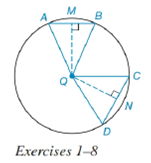Chapter 6.4, Problem 2E### Elementary Geometry for College St...

6th Edition
Daniel C. Alexander + 1 other
ISBN: 9781285195698

#### Solutions

Chapter
Section### Elementary Geometry for College St...

6th Edition
Daniel C. Alexander + 1 other
ISBN: 9781285195698
Textbook Problem
1 views

# In Exercises 1 to 8, use the figure provided.If m C D ⌢ < m A B ⌢ , write an inequality that compares C D and A B .To determine

To write:

An inequality that compares CD and AB.

Explanation

Given:

mCD<mAB and the figure given below

Theorem:

In a circle (or in congruent circles) containing two unequal minor arcs, the greater minor arc corresponds to the longer of the chords related to these arcs.

Calculation:

Using the theorem.

Since the arcs are unequal i.e. mCD<mAB and from the given figure

### Still sussing out bartleby?

Check out a sample textbook solution.

See a sample solution

#### The Solution to Your Study Problems

Bartleby provides explanations to thousands of textbook problems written by our experts, many with advanced degrees!

Get Started